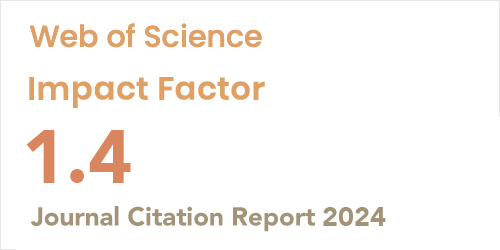ISSN: 2148-7847
Volume: 9 Issue: 3 Year: 2023Statistical analysis of the solar diffuse fraction radiation using regression analysis of longitudinal data in India
1Laboratory of Applied Research in Hydraulics, University of Mustapha Ben Boulaid-Batna,16010, Algeria
2Department of Economics, Mohamed Boudiaf University, M’sila, 28000, Algeria
3Computer Science and Statistics Department, Universidad Rey Juan Carlos Móstoles, Mostoles, 28933, Madrid
J Ther Eng 2023; 3(9): 776-785 DOI: 10.18186/thermal.1300542

#### Abstract

In this study, the validity of the estimation of a single regression equation for the diffuse frac-tion across 22 stations in India using the two parameters: the clearness index and the sunshine ratio is tested. The homogeneity test based on Fisher’s statistics was applied to test the homo-geneity of the estimated parameters across all stations. The results showed that the p-value at the level of 5% for each model is smaller than 0.05, indicating that all stations were heteroge-neous. The Hierarchical Cluster Analysis (HCA) was used to classify the data into homoge-nous clusters. The results of HCA indicated that the longitudinal data were divided into four main clusters. For each cluster, the regression analysis was applied based on the longitudinal data then, the fixed effects model (FEM) and the random-effects model (REM) were used for the evaluation. Further, the Hausman test was applied to choose between the fixed effects model and the random-effects model. Finally, the results showed that the four best regression models were found for the selected stations in the study area.